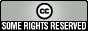Improving economics teaching and learning for over 20 years

Surprise exam questions in Markets, Games and Strategic Behaviour

Todd Kaplan, University of Exeter/ University of Haifa
Put online February 2010

The following are bonus questions extracted from exams shared through the TRUE wiki for Experimental Economics. This text is licensed as Creative Commons, some rights reserved.

• Guess a number between 0 and 100. The closest person to the (2/3 times the average number) wins a bonus of 1% on the final. Ties will be broken randomly.
• Outcome: Average was 28:6. Two-thirds of this is 19:1. Winning guess was 21.
• Guess a number between 0 and 100. The closest person to the 33.333+(2/3 times the average number) wins a bonus of 1% on the final. Ties will be broken randomly.
• Outcome: the winning number was 75. The equilibrium here is 100.
• You will be matched with 4 other students. Tell me how much of a bonus you would like on the exam in percentage terms. Please write down a number 0 and 100 (fractions permitted). I will give the bonus to the student with the lowest request. Ties will be broken randomly.

See the full exam papers.

Authors:Except where stated, resources on this page are available under a Creative Commons by-nc licence.